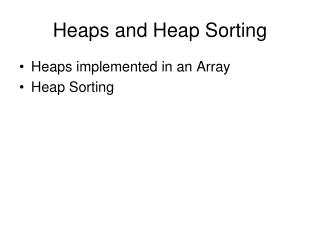DownloadDownload PresentationHeaps and Heap Sorting

# Heaps and Heap Sorting

Télécharger la présentation## Heaps and Heap Sorting

- - - - - - - - - - - - - - - - - - - - - - - - - - - E N D - - - - - - - - - - - - - - - - - - - - - - - - - - -
##### Presentation Transcript

1. Heaps and Heap Sorting • Heaps implemented in an Array • Heap Sorting

2. Implementing Heaps in an Array • Two of the programming steps for a heap using links were complicated • Finding the next parent for an add • Finding the new value for next after a remove • Those steps are trivial for a heap in an array • If the index 0 is the root and “next” is the index for a reference to the next open space: • After adding an element, just increment next • To remove an element, just decrement next

3. Implementing Heaps in an Array • The class header, attributes, and constructor: public class ArrayHeap<T extends Comparable> ... { // not shown as a subclass like in textbook private static final int DEFAULT_CAPACITY = 50; protected int count; protected T[] tree; public ArrayHeap() { count = 0; tree = (T[]) new Object[DEFAULT_CAPACITY]; }

4. Implementing Heaps in an Array • The add method: public void addElement(T obj) { if (count == tree.length) expandCapacity(); // same as for any array tree[count++] = obj; if (count > 1) heapifyAdd(); }

5. Implementing Heaps in an Array • The heapifyAdd helper method private void heapifyAdd() { int next = count – 1; T temp = tree[next]; // pick up new value while ((next != 0) && // move up the tree as needed temp.compareTo(tree[(next-1)/2] < 0 { tree[next] = tree[(next-1)/2]; //move parent down next = (next – 1)/2; } tree[next] = temp; // (re)insert new value }

6. Implementing Heaps in Arrays • The removeMin method: public T removeMin() ... { // check for empty heap not shown T minElement = tree; // start at the root tree = tree[count–1]; heapifyRemove(); tree[--count] == null; // kill stale reference return minElement; }

7. Implementing Heaps in Arrays • The heapifyRemove method (My version): private void heapifyRemove() { int node = 0; T temp = tree[node]; int next = 0; do { if (node != 0) { // skip until second+ pass tree[node] = tree[next]; node = next; }

8. Implementing Heaps in an Array • The heapifyRemove method (continued): int left = 2 * node + 1; int right = 2 * (node + 1); if(tree[left] == null && tree[right] == null) next = count; // force end of loop else if (tree[right] == null || tree[left].compareTo(tree[right]) < 0) next = left; else next = right; } while (next < count && tree[next].compareTo(temp) < 0 ); tree[node] = temp; }

9. Implementing Heaps in Arrays • I didn’t like the repetition of 8 lines of code in the initialization before the while loop and as 80% of the code in the body of the loop • Whenever you see that situation in code, it is a clue that a do-while loop might be better • I rewrote the heapifyRemove method as a do-while loop instead of the textbook code that uses a while loop - both code versions perform the same steps in the same order

10. Heap Sorting • If we have a method with a parameter that is an array of type T to be sorted, it can be written to use a heap instead of one of the in-place array sorts we studied in lecture 19 • We can take each element from the array and put it in a heap • Then loop removing the min from the heap and putting each element back into the array in order

11. Heap Sorting // only this method needs to be shown for the example public void heapsort(T[] data) { HeapADT<T> temp = new ArrayHeap<T>(); // copy the array into the heap for (T datum : data) // use a for-each loop temp.addElement(datum); // place the sorted elements back into the array int count = 0; while(!temp.isEmpty()) data[count++] = temp.removeMin(); } // temp goes out of scope

12. Heap Sorting Performance • The performance is 2*N*log N or O(N logN) • That is the same as quicksort or merge sort • It uses the same amount of extra memory as the merge sort algorithm